# Working in the Cartesian plane

### Term 1 starting in week 7 :: Estimated time: 3 weeks

• Work with coordinates in all four quadrants
• Identify and draw lines that are parallel to the axes
• Recognise and use the line $$y = x$$
• Recognise and use lines of the form $$y = kx$$
• Link $$y = kx$$ to direct proportion problems
• Recognise and use lines of the form $$y = x + a$$
• Explore graphs with negative gradient ($$y = -kx, y=a-x, x+y=a$$)
• Link graphs to linear sequences
• Plot graphs of the form $$y = mx + c$$

For higher-attaining pupils:

• Explore the gradient of the line $$y = kx$$
• Explore non-linear graphs
• Find the midpoint of a line segment

This page should remember your ticks from one visit to the next for a period of time. It does this by using Local Storage so the information is saved only on the computer you are working on right now.

## Lesson Starters

Here are some suggestions for whole-class, projectable resources which can be used at the beginnings of each lesson in this block.

### 1st Lesson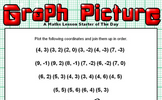#### Graph Picture

Plot the given coordinates then join them up to form a picture.

### 2nd Lesson#### Middle of Centres

The blue point is exactly in the middle of two red points. What are their coordinates?

### 3rd Lesson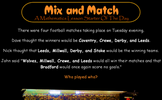#### Mix and Match

Work out which team played which from the information given.

### 4th Lesson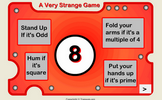#### A Very Strange Game

Four different actions depending on the number which appears.

### 5th Lesson#### Two Twins and Tim

A tricky problem set on a coordinate grid.

### 6th Lesson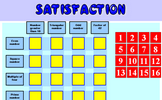#### Satisfaction

Rearrange the numbers, row and column headings so that this table is mathematically correct.

### 7th Lesson#### Sum Equals Product

What are the numbers if their sum equals their product?

### 8th Lesson#### Noodlewhack

Divide one whole number by another on your calculator. If the answer has one digit repeated but no other digits you have a Noodlewhack.

### 9th Lesson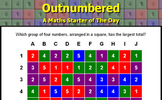#### Outnumbered

Which group of four numbers, arranged in a square, has the largest total?

Some of the Starters above are to reinforce concepts learnt, others are to introduce new ideas while others are on unrelated topics designed for retrieval practice or and opportunity to develop problem-solving skills.

White Rose ResourcesEnd of block assessments provide a quick progress check at the end of each block of learning to make sure students have understood the content covered. This Scheme of Learning was produced by White Rose Maths and is used here with permission granted on 30th June 2021.For All: